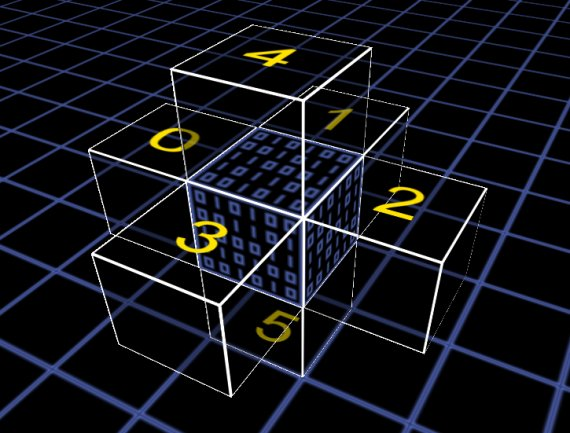1. # PlaceVoxel

## Description

bool PlaceVoxel(integer Location, integer VoxelType)

Get a voxel in the robot storage unit and place it at the specified location. You can place a voxel in the 6 voxels which are directly adjacent. The requested material must be available in the robot's storage. This function can only be used in Voxel_Step().

## Parameters

VoxelType : The VoxelType code of the material you want to place. The requested material must be available in the robot's storage.

Location : The position of the voxel to grab in "position code" notation.

0 =  { 0, 0, 1 } = Front of the robot.
1 =  { 1, 0, 0 } = Right of the robot.
2 =  { 0, 0, -1} = Back of the robot.
3 =  {-1, 0, 0 } = Left of the robot.
4 =  { 0, 1, 0 } = Above the robot.
5 =  { 0,-1, 0 } = Under the robot.## Return Value

A boolean number. It will be true if the function placed a voxel successfully or false otherwise.

## Example of use

Put the robot on the ground in the blue zone and it will mine materials and build structures.

```// Listing #1: Automatic Builder
y <- 0;
state <-0;
count <-0;
floors<-0;

function Voxel_Step()
{
local i;

switch (state)
{
case 0: for(i=0;i<6;i++) PickVoxel(i);
Move(5);
y++;
if (y > 64) state = 1;
break;
case 1: Move(4);
y--;
if (y<1) {state = 2; }
break;
case 2: Move(0);
state = 3;
break;
case 3: Move(1);
state = 4;
break;
case 4: Move(4);
state = 5; count = 3; floors = 0;
break;
case 5: if (floors >9 ) {state = 10; break; }
if (!PlaceVoxel(5,1))
{
if (floors > 5) state = 10;
else            state = 13;
break;
}
Move(2);
count--;
if (count <=0) { state = 6; count = 3; }
break;
case 6: PlaceVoxel(5,1);
Move(3);
count--;
if (count <=0) { state = 7; count = 3; }
break;
case 7: PlaceVoxel(5,1);
Move(0);
count--;
if (count <=0) { state = 8; count = 3; }
break;
case 8: PlaceVoxel(5,1);
Move(1);
count--;
if (count <=0) { state = 9; }
break;
case 9: Move(4);
state=5;count=3; floors++;
break;
case 10:Move(3);
state=11; count=4;
break;
case 11:Move(0);
count--;
if (count <=0) { state = 12; }
break;
case 12:if (!Move(5)) state = 0;
break;
case 13:break;
}
}

```